# 相变蓄冷保温箱的模拟仿真与实验Numerical Simulation and Experimental Investigation of Storage Box Using Phase Change Materials

DOI: 10.12677/MOS.2021.101018, PDF, HTML, XML, 下载: 49  浏览: 91

Abstract: An insulated box was made from a new type of low temperature phase change material (PCM)., which is to test the thermal performance of this new type PCM and find the melting law of the PCM in this box . The no-load experiment was carried out with the self-made box. The results show that the proportion of the PCM in the upper layer can be increased because of the rapid melting of the PCM in the upper layer. The heat transfer model can ensure the cold storage time to meet people’s needs and also can be applied to the cold chain logistics system. ANSYS Fluent software was used to simulate the temperature variation of the box. The simulation results show that the bottom tem-perature curves are in agreement with the experimental results and consistent. There is a big dif-ference in the intermediate temperature because there is natural convection of hot air in the reali-ty rising so that the intermediate temperature is higher than the bottom temperature. The PCM’s melting inside the storage box usually starts from the corner and the corner structure needs to be optimized. Due to the presence of heat leakage, the experimental temperature is seriously strati-fied. However, in the simulation, the temperature distribution is uniform and there is basically no stratification because of idealized conditions and no heat leakage.

1. 引言

2. 保温箱设计与实验

2.1. 保温箱的设计计算Table 1. Coefficient of insulation material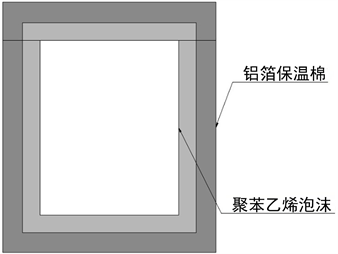Figure 1. Section of box (vertical section)

${S}_{o}=2\ast \left({a}_{o}\ast {b}_{o}+{a}_{o}\ast {b}_{o}+{b}_{o}\ast {h}_{o}\right)$ (1)

${S}_{i}=2\ast \left({a}_{i}\ast {b}_{i}+{a}_{i}\ast {b}_{i}+{b}_{i}\ast {h}_{i}\right)$ (2)

$S=\sqrt{{S}_{o}\ast {S}_{i}}$ (3)

${k}_{2}=1/\left(\frac{{\delta }_{1}}{{\lambda }_{1}}+\frac{{\delta }_{2}}{{\lambda }_{2}}\right)$ (4)

${\delta }_{1}$${\delta }_{2}$ 分别为泡沫层和保温棉的厚度 m， ${\lambda }_{1}$${\lambda }_{2}$ 分别为泡沫层和保温棉层的导热系数， w/(m·k)。

${k}_{o}=1/\left(\frac{1}{{k}_{1}}+\frac{1}{{k}_{2}}+\frac{1}{{k}_{3}}\right)$ (5)

$\Delta T={T}_{o}-{T}_{i}$ (6)

${T}_{o}$ , ${T}_{i}$ 分别为箱外环境温度和箱内冷藏温度，℃。

$Q=3600t{K}_{o}A\Delta T$ (7)

Q为散热量J； ${K}_{o}$ 为传热系数w/(m2·k) ；t为保冷时间h；A为传热面积m2$\Delta T$ 为传热温差℃；

$m=\frac{Q}{H}$ (8)

m——蓄冷剂质量g；

H——相变蓄冷材料相变潜热J/g；本实验所用蓄冷剂的相变潜热为222.7 J/g。

2.2. 实验过程

2.2.1. 实验仪器

2.2.2. 实验步骤

3. 模拟

3.1. 模型的建立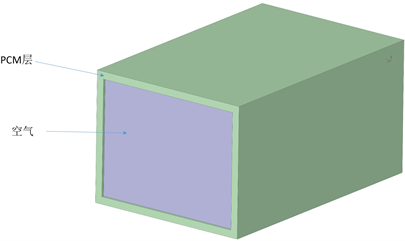Figure 2. The diagram of numerical model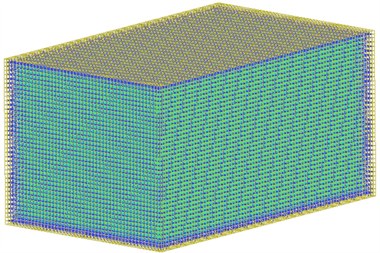Figure 3. The diagram of grid

3.2. 数学模型及基本假设

$\frac{\partial \left(\rho \varphi \right)}{\partial t}+div\left(\rho V\varphi \right)=div\left({\Gamma }_{\varphi }grad\varphi \right)+{S}_{\varphi }$ (9)

3.3. 边界条件与求解

${G}_{r}=\frac{g{\alpha }_{v}\Delta t{l}^{2}}{{v}^{2}}$ (10)

(10)式中， ${\alpha }_{v}$ 为体胀系数，本材料体胀系数为0.84 m3/kg；l表示长度特征尺度，本例为0.14 m； $\Delta t$ 温差，本例最大温差不超过20℃；g重力加速度，9.81 m/s2$\nu$ 运动粘度系数，取15 * 10−6

$\beta =\left\{\begin{array}{l}0,\text{\hspace{0.17em}}\text{\hspace{0.17em}}\text{\hspace{0.17em}}\text{\hspace{0.17em}}\text{\hspace{0.17em}}\text{\hspace{0.17em}}\text{\hspace{0.17em}}\text{\hspace{0.17em}}\text{\hspace{0.17em}}\text{\hspace{0.17em}}\text{\hspace{0.17em}}\text{\hspace{0.17em}}\text{\hspace{0.17em}}\text{\hspace{0.17em}}\text{ }T<{T}_{S}\\ \frac{T-{T}_{S}}{{T}_{L}-{T}_{S}},\text{\hspace{0.17em}}\text{\hspace{0.17em}}{T}_{S}{T}_{L}\end{array}$ (11)

4. 结果与分析

4.1. 实验结果分析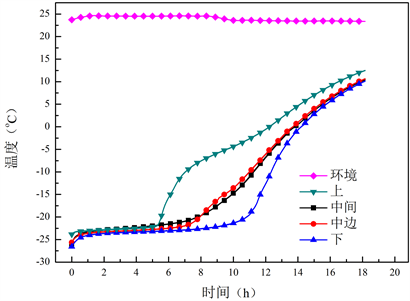Figure 4. Temperature curve of no load experiment with 0.836 kg PCM

4.2. 模拟结果分析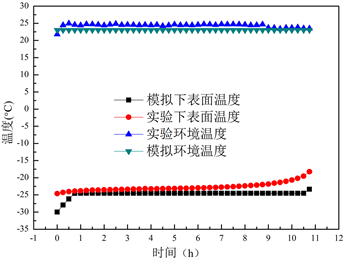(a)(b)

Figure 5. Experiment and simulation’s temperature contrast diagramFigure 6. Simulation liquid fraction diagram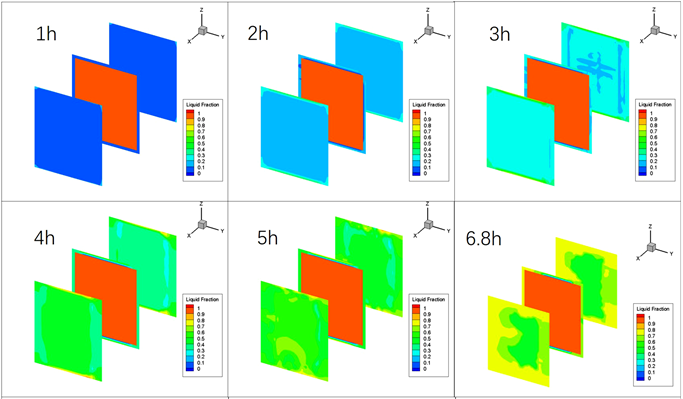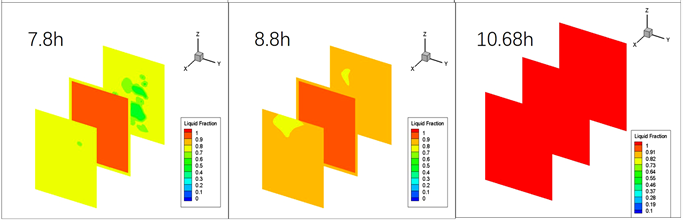Figure 7. Actual change diagram of liquid fraction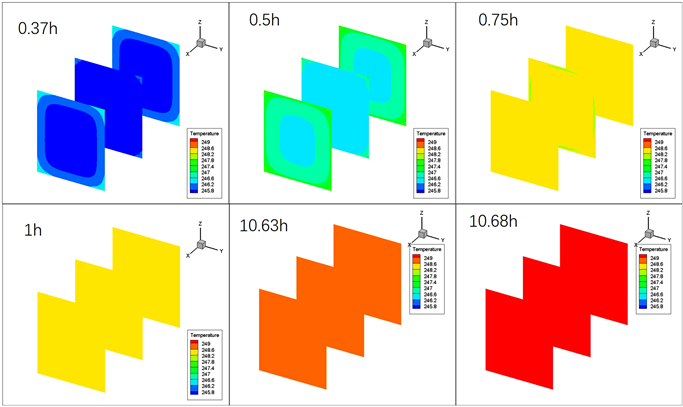Figure 8. Temperature change diagram of simulation

5. 结语

1) 加装蓄冷材料会延长保冷时间，用传热模型计算所得的蓄冷剂质量将保温箱蓄冷时间维持在8小时左右，能够满足人们日常所需。

2) 经试验验证，保温箱底面保冷实验的温度模拟值与试验值随时间变化规律基本吻合，说明所建立的模型比较可靠。但有2℃左右的温差，这是因为实验环境温度不能完全稳定，实验一开始不能达到密封性要求，其次因为模型的简化，忽略掉了自封袋的不均匀性。

3) 通过对蓄冷式冷藏箱降温过程的数值模拟发现：相变材料从边角开始相变，分层可以优化边角。模拟与实验都验证蓄冷材料对保温箱的保冷具有主要的作用，可以将食品储存在保温箱环形区域内。采用fluent对蓄冷式冷藏箱降温过程中温度场的数值模拟具有一定的科学性，有助于认清温度场分布规律，为蓄冷式冷藏箱降温过程的参数优化提供参考。

  Mehling, H. and Cabeza, L.F. (2008) Heat and Cold Storage with PCM. Springer, Berlin, Heidelberg. https://doi.org/10.1007/978-3-540-68557-9  杨天润, 孙锲, Ronald, W., 程林. 相变蓄冷材料的研究进展[J]. 工程热物理学报, 2018, 39(3): 567-573.  黄艳, 章学来. 蓄冷技术在食品冷链物流中的研究进展[J]. 包装工程, 2015, 36(15): 23-29.  贾蒲悦, 武卫东, 王益聪. 新型0℃相变蓄冷材料制备及蓄冷特性[J]. 化工进展, 2019, 38(6): 2862-2869. http://dx.chinadoi.cn/10.16085/j.issn.1000-6613.2018-1611  陈文朴, 章学来, 黄艳, 任迎蕾, 丁锦宏. 甲酸钠低温相变材料的研制及其在蓄冷箱中的应用[J]. 制冷学报, 2017, 38(1): 68-72. http://dx.chinadoi.cn/10.3969/j.issn.0253-4339.2017.01.068  Ahmed, M., Meade, O. and Medina, M.A. (2010) Reducing Heat Transfer across the Insulated Walls of Refrigerated Truck Trailers by the Application of Phase Change Materials. Energy Conversion and Management, 51, 383-392. https://doi.org/10.1016/j.enconman.2009.09.003  Liu, M., Saman, W. and Bruno, F. (2012) Development of a Novel Refrigeration System for Refrigerated Trucks Incorporating Phase Change Material. Applied Energy, 92, 336-342. https://doi.org/10.1016/j.apenergy.2011.10.015  徐笑锋, 章学来, Munyalo, J.M., 李玉洋, 陈跃, 陈启杨, 等. 十水硫酸钠相变蓄冷保温箱保冷特性的试验研究[J]. 农业工程学报, 2017, 33(22): 308-314. http://dx.chinadoi.cn/10.11975/j.issn.1002-6819.2017.22.040  刘伟, 杨洲, 段洁利, 赵文峰, 邱汉. 蓄冷式冷藏箱降温过程的数值模拟及试验验证[J]. 华南农业大学学报, 2019, 40(4): 119-125. http://dx.chinadoi.cn/10.7671/j.issn.1001-411X.201810001  田津津, 张哲, 王怀文, 王飒飒, 李立民, 郭永刚. 蓄冷板释冷过程的数值模拟和实验研究[J]. 制冷学报, 2016, 37(3): 29-34. http://dx.chinadoi.cn/10.3969/j.issn.0253-4339.2016.03.029  赵慧妍, 付志强, 王岩, 吕洪利, 纪秋平, 康勇刚. 基于Fluent的XPS保温箱温度场数值模拟方法分析[J]. 包装工程, 2018, 39(19): 105-109. http://dx.chinadoi.cn/10.19554/j.cnki.1001-3563.2018.19.019  朱文娴, 柯元裕, 卢立新, 王力, 贾代涛. 食品冷藏保温箱温度场模拟与实验验证[J]. 包装工程, 2018, 39(23): 39-74. http://dx.chinadoi.cn/10.19554/j.cnki.1001-3563.2018.23.012  潘欣艺, 王家俊, 王冬梅. 蓄冷剂摆放位置对保温箱中温度场的影响[J]. 包装工程, 2018, 39(3): 77-82. http://dx.chinadoi.cn/10.19554/j.cnki.1001-3563.2018.03.015  吕传超, 崔振科, 王乐. 冰箱冷藏室温度场和流场的数值模拟与结构优化[J]. 制冷技术, 2015, 35(5): 56-61. http://dx.chinadoi.cn/10.3969/j.issn.2095-4468.2015.05.204  吴肖骏, 胡桂林, 周春豪, 余时帆. 冷冻海鲜产品保温箱结构设计与优化[J]. 包装工程, 2018, 39(3): 72-76. http://dx.chinadoi.cn/10.19554/j.cnki.1001-3563.2018.03.014  余永涛. 潜热型控温包装相变传热过程模拟与分析[D]: [硕士学位论文]. 无锡: 江南大学, 2016: 1-25.  菅宗昌, 潘瞭, 卢立新. 一种典型潜热型控温包装的有限元分析[J]. 包装工程, 2014, 35(9): 14-17.  Lu, W., Liu, G.Z., Xing, X.H. and Wang, H. (2019) Investigation on Ternary Salt-Water Solutions as Phase Change Materials for Cold Storage. Energy Procedia, 158, 5020-5025. https://doi.org/10.1016/j.egypro.2019.01.662  潘嘹, 卢立新, 王军. 控温包装控温时间预测模型研究[J]. 包装工程, 2014, 35(5): 27-30, 136.  杨世铭, 陶文铨. 传热学[M]. 北京: 高等教育出版社, 2006.  李晓燕, 王雪雷, 苗馨月, 陈杰, 闫淑晴. 冷链蓄冷保温箱性能优化数值模拟研究[J]. 节能技术, 2019, 37(213): 87-90. http://dx.chinadoi.cn/10.3969/j.issn.1002-6339.2019.01.018  沈海英. 复合相变蓄冷材料的热物性及其应用在冰箱内的数值模拟[D]: [硕士学位论文]. 重庆: 重庆大学. 2008: 35-50.